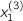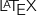# CVXGEN: Code Generation for Convex Optimization

## Expressions

Parameters, variables, numbers and dimensions are combined to create expressions, which can then be used in the objective and constraints.

You can use addition, subtraction, negation, multiplication, transpose, and scalar parameter division. You can also combine these operations with various functions.

Examples, assuming previously defined symbols and valid dimensions:

A*x + b

-x

negation

p'*x

transpose and multiplication

(p + z)'*x

parentheses, transpose and multiplication

p'*x

accessing an indexed variable, if previously defined (equivalent to)

(1/a)*A*z

scalar division (a must be a scalar parameter or a dimension)

z + z

accessing the first and third elements of a vector (equivalent to)

x

accessing the first element of an indexed variable (equivalent to)

### Notes

• Be cautious when indexing variables or parameters; the same syntax is used both for accessing indexed variables or parameters, and for accessing the elements of parameters or variables. In general, mirror the relevant mathematics in your problem statement, and check theoutput provided by CVXGEN to ensure you are getting what you want.

• You cannot place a vector, or a scalar optimization variable, in the denominator of a scalar ratio.

• Operation order mirrors mathematics. Note: ‘/’ binds more tightly than any other operator, so 1/2*a means (1/2)*a. Check CVXGEN'soutput if unsure.